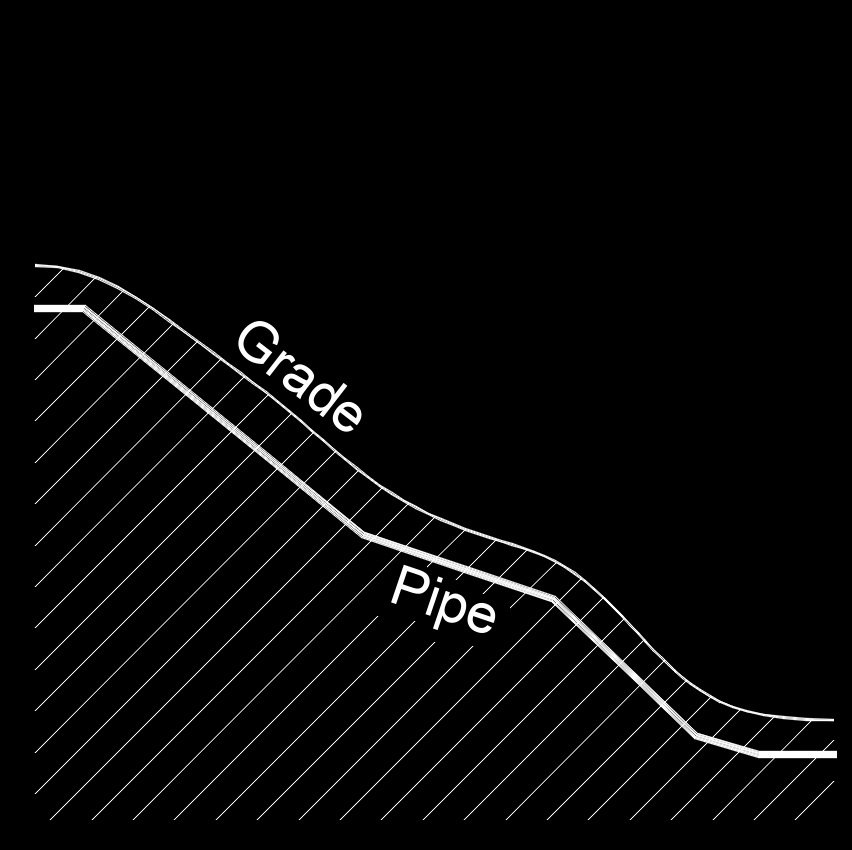Written by Jerry Ratzlaff on . Posted in Fluid DynamicsHead loss, abbreviated as $$h_l$$, is a pressure loss due to the resistance of the fluid and obstructions along the way in a pipe.  There are a number of factors that affect head loss such as:

• flow rate
• corrosion and scale deposits
• fittings (bends, elbows, exits, expanders and reducers, tees)
• inside diameter of the pipe
• roughness of the pipe wall
• viscosity of the liquid
• length of the pipe
• straightness of the pipe
• valves

Major head loss, abbreviated as $$h_{l-major}$$, is the pressure drop caused by friction loss.  It does not matter if the pipe is horizontal, vertical or on an incline.

 $$\large{ h_{l-major} = f \; \frac{l}{d} \; \frac{v^2}{2\;g} }$$

### Where:

$$\large{ h_{l-major} }$$ = major head loss

$$\large{ f }$$ = friction factor

$$\large{ g }$$ = gravitational constant (32.2 ft/sec)

$$\large{ l }$$ = lenght of pipe

$$\large{ d }$$ = pipe inside diameter

$$\large{ v }$$ = velocity of flow

Minor head loss, abbreviated as $$h_{l-minor}$$, is the pressure drop caused by fittings and valves.

 $$\large{ h_{l-minor} = K \; \frac{v^2}{2 \; g} }$$

### Where:

$$\large{ h_{l-minor} }$$ = minor head loss

$$\large{ g }$$ = gravitational constant (32.2 ft/sec)

$$\large{ K }$$ = loss coefficient

$$\large{ v }$$ = velocity of flow